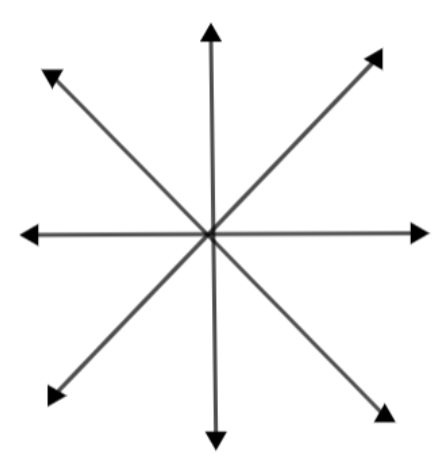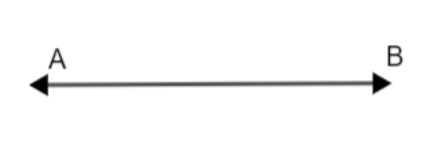Courses
Courses for Kids
Free study material
Free LIVE classes
MoreLIVE
Join Vedantu’s FREE Mastercalss

# How many lines can pass through two given points?Verified
271.2k+ views
Hint: Infinite number of lines can pass through a single point. Similarly, an infinite number of curves can pass through 2 points, which are not straight lines. Only 1 straight line can pass through 2 points.

Let us consider the 2 points as A and B.
Now, infinite lines can pass through the point A as shown below.Similarly, infinite lines can pass through point B.
Now, Let's take two points A and B together will have one line passing through it.Only through Point A $\to$ Infinite lines.
Only through Point B $\to$ Infinite lines.
Through A and B $\to$ 1
Number of straight lines that can pass through 2 points A and B = 1.

Note:
Let us consider a case of non-collinear points. If we have been given 4 points and need to find how many lines can pass through these points.
The method is to find the number of straight lines that can be formed.3 Lines 5 Lines 6 Lines
$\therefore$(4 - 1) (4 - 1) + (4 - 2) (4 - 1) + (4 - 2) + (4 - 3) + (4 - 4)
$\therefore$They are of the form:
\begin{align} & h=\sum\limits_{i=1}^{n}{\left( n-i \right)}=\left( n-1 \right)+\left( n-2 \right)+\left( n-3 \right)+.....+\left( n-n \right) \\ & =\sum\limits_{i=1}^{n}{n}-\sum\limits_{i=1}^{n}{i}\Rightarrow L={{n}^{2}}-\dfrac{n\left( n+1 \right)}{2}=\dfrac{2{{n}^{2}}-{{n}^{2}}-n}{2} \\ & L=\dfrac{{{n}^{2}}-n}{2}=\dfrac{n\left( n-1 \right)}{2} \\ \end{align}
$\therefore L=\dfrac{n\left( n-1 \right)}{2}$, where L = number of lines.
So, for 4 points, n=4,
$L=\dfrac{4\left( 4-1 \right)}{2}=\dfrac{4\times 3}{2}=6$lines.
Where n=1, $L=\dfrac{1\left( 1-1 \right)}{2}=\dfrac{0}{2}$i.e. Infinite number of lines.
Where n=2, $L=\dfrac{2\left( 2-1 \right)}{2}=1$etc.

Last updated date: 29th Sep 2023
Total views: 271.2k
Views today: 5.71k